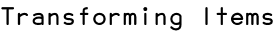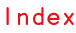RSS

This tutorial explains how to move, scale and rotate items in your Illustrator document using Scriptographer.

## Changing the Position of an Item

Using Scriptographer you can move an item around in the document by changing its item.position property. This moves the item by its center point.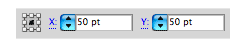For example let's make a path and then move it to another position:

```var circlePath = new Path.Circle(new Point(50, 50), 25);
circlePath.position = new Point(100, 100);
```

Let's quickly go through the code above. In the first line we create a circle shaped path with its center point at {x: 50, y: 50} with a radius of 25 pt. In the second line we change the position (the center point) of the circle shaped path to {x: 100, y: 100}

We can also move an item by a certain amount using the += operator.

The following example creates the same path as above, but instead of moving it to an absolute position, moves it 10pt to the right and 20pt down.

```var circlePath = new Path.Circle(new Point(50, 50), 25);
circlePath.position += new Point(10, 20);
```

## The Bounding Rectangle of an Item

When you select an item in Illustrator and select the transform tool it draws a rectangle around the item which describes its boundaries: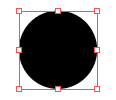In Scriptographer we can find out the dimensions of this bounding rectangle by looking at the item.bounds property of item.

The item.bounds property is a Rectangle. Rectangles are described in detail in the Point, Size and Rectangle tutorial.

For example if want to know the width and height of an item, we can query the item.bounds property for width and height:

```var circlePath = new Path.Circle(new Point(50, 50), 25);
print(circlePath.bounds.width); // 50
print(circlePath.bounds.height); // 50
```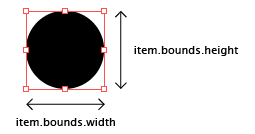We can also find the position of the corner points of the bounding rectangle:

```var circlePath = new Path.Circle(new Point(50, 50), 25);
print(circlePath.bounds.topLeft); // { x: 25.0, y: 25.0 }
print(circlePath.bounds.topRight); // { x: 75.0, y: 25.0 }
print(circlePath.bounds.bottomRight); // { x: 75.0, y: 75.0 }
print(circlePath.bounds.bottomLeft); // { x: 25.0, y: 75.0 }
```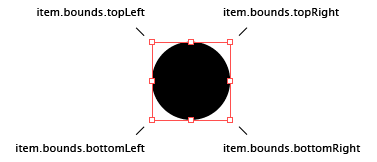## Scaling Items

In Illustrator you can scale an item by selecting it in the document, selecting the Scale Tool in the tool palette and clicking and dragging in the document to scale it. You can also change the center point of the scaling operation by clicking in the document.

Using Scriptographer you can also scale items. To scale both the width and height of an item by the same amount, you can call the item.scale(scale) of the item with a value between 0 and 1.

Let's make a path and then scale it by 50%:

```var circlePath = new Path.Circle(new Point(50, 50), 25);
circlePath.scale(0.5);
```

## Scaling an Item Around a Center Point

By default the item.scale(scale) function scales an item around its center point. If you want to scale around a specific position, you can pass the scale function an optional center point: item.scale(scale, point).

Let's make a path and then scale it by 50% from {x: 0, y: 0}:

```var circlePath = new Path.Circle(new Point(50, 50), 25);
circlePath.scale(0.5, new Point(0, 0));
```

Since the corner positions of the item.bounds bounding rectangle are also points, we can also pass them to the item.scale(scale, point) function to act as a center point for the scale transformation.

For example, if we would want to scale an item by 50% using the top right position of the bounding rectangle:

```var circlePath = new Path.Circle(new Point(50, 50), 25);
circlePath.scale(0.5, circlePath.bounds.topRight);
```

Items can also be scaled with different horizontal and vertical scales. To do this we pass two numbers to scale using item.scale.

For example, we can create a path and scale it horizontally by 25% and vertically by 150%:

```var circlePath = new Path.Circle(new Point(50, 50), 25);
circlePath.scale(0.25, 1.5);
```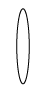## Rotating Items

To rotate an item in the document we call the item.rotate(angle) function and pass it the angle we want to rotate by in degrees. This will rotate the item by the angle in a clockwise direction.

Now, let's create a rectangle shaped path and rotate it by 45 degrees:

```var path = new Path.Rectangle(new Point(50, 50), new Size(100, 50));
path.rotate(45);
```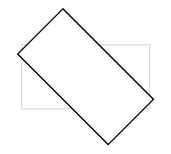To rotate in a counter-clockwise direction we pass a negative angle to the item.rotate(angle) function:

```var path = new Path.Rectangle(new Point(50, 50), new Size(100, 50));
path.rotate(-45);
```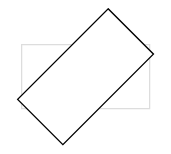In the same way as you can pass a point to the scale function to scale around, you can also pass a point to the rotate function to rotate around.

For example, let's rotate around the bottom left point of the bounding rectangle:

```var path = new Path.Rectangle(new Point(50, 50), new Size(100, 50));
path.rotate(-45, path.bounds.bottomLeft);
```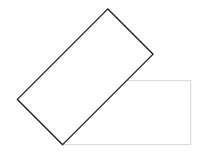Lets write a more advanced script that clones an item multiple times in a loop and rotates the clones by different angles.

Cloning (copying) items is described at the end of the Working with Items tutorial.

```var circlePath = new Path.Circle(new Point(150, 150), 25);
var clones = 30;
var angle = 360 / clones;

for(var i = 0; i < clones; i++) {
var clonedPath = circlePath.clone();
clonedPath.rotate(angle * i, circlePath.bounds.topLeft);
};
```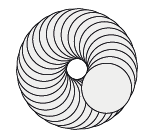Let's go through this script line by line.

First we create the path that we will be cloning and we make a variable called clones where we store the amount of clones that we want:

```var circlePath = new Path.Circle(new Point(150, 150), 25);
var clones = 30;
```

In the third line, we make a variable called angle and pass it 360 degrees (a full rotation) divided by the amount of clones we will be making:

```var angle = 360 / clones;
```

Next, we loop the amount of times we defined in the clones variable:

```for (var i = 0; i < clones; i++) {
```

Basically what this line does is make a variable called i and start it with 0. Then it executes the block of code that is in between the { } brackets. After executing, it adds 1 to i and compares i to the clones variable. If i is still smaller then the clones variable, it executes the code block again.

Within the loop we clone the path using item.clone() and rotate it by angle * i from the top left point of its bounding rectangle. This means that when i is 0, the cloned path is rotated by 0 * angle and when i is 5 it rotates the cloned path by 5 * angle:

```	var clonedPath = circlePath.clone();
clonedPath.rotate(angle * i, circlePath.bounds.topLeft);
```